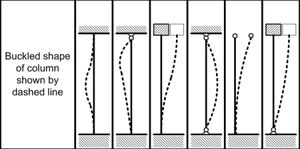This section first calculates the critical slenderness ratio, Cc for the material whose properties are entered, compares this Cc value with the slenderness ratio of the column and if the slenderness ratio is greater than Cc, the buckling load is calculated by the Euler formula, whereas if the slenderness ratio is less than Cc, the Johnson formula is used.